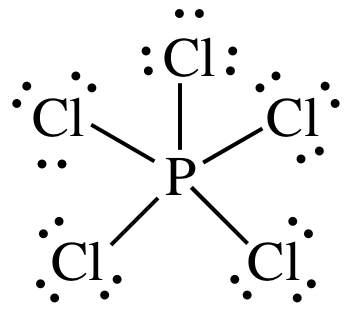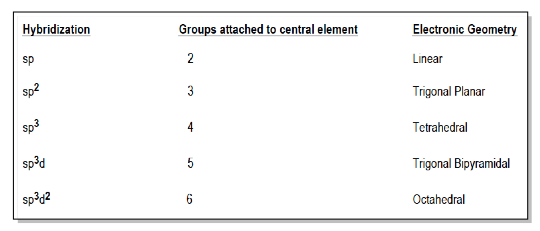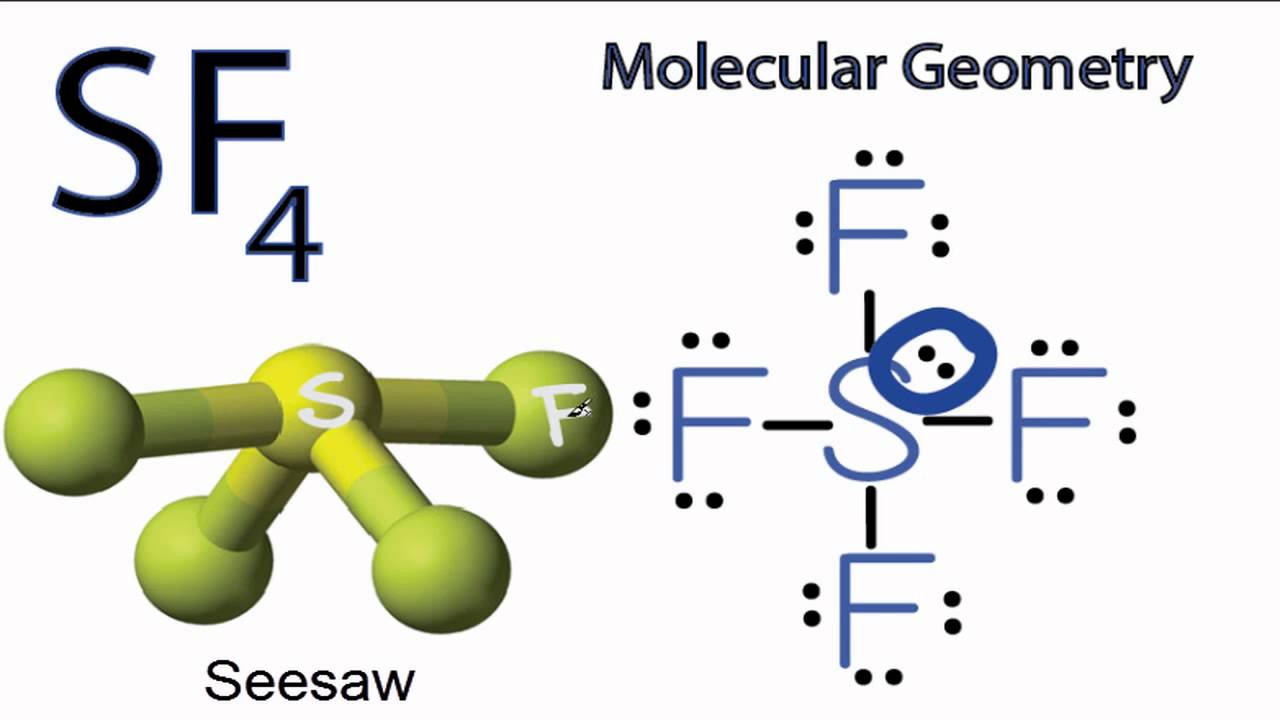Resources:

Have you found videos, websites, or explanations that helped you understand this chapter? Let us know and we'll add them to "Resources" part of this page for other students to use.

Question 1

Determine the geometry or shape of a MgH2 molecule. Check those statements which are correct.

Select one or more:Explanation

MgH2 : 2+ 2= 4 electrons

Mg : 2 bonds = 2 steric number

2 bonds +  0 lone pairs  = linear

Question 2

Determine the geometry or shape of a BCl2- ion. Hint in general feedback

Select one or more:Explanation

BCl2: 3 + ( 2*7) + 1 =18 electrons

B: 2 bonds + 1 lone pair = 3 steric number

2 bonds + 1 lone pair  = bent

Question 3

Determine the geometry or shape of a SiF4 molecule. Hint in general feedback
Select one or more:ExplanationQuestion 4

Determine the geometry or shape of a CO2 molecule. Hint in general feedback

Select one or more:Question 5

Draw the Lewis structure and shape for the phosphine, PH3. Which of the following statements are correct.

Select one or more:Explanation

PH3 : 5+ ( 1 * 3) = 8

C: 3 bonds + 1 lone pairs = 4 steric number

3 bonds + 1 lone pair  = trigonal pyramidalQuestion 6

Draw the Lewis structure and shape for PCl5. Which of the following statements are correct.

Select one or more:Explanation

PCl 5 : 5 +( 7*5) = 40

P: 5 bonds + 0 lone pairs = 5 steric number

5 bonds + 0 lone pair  =trigonal bipyramidalQuestion 7

Draw the Lewis structure and shape for SF6. Which of the following statements are correct.

Select one or more:Explanation

SF6 : 6 +( 6*7) = 48

P: 6 bonds + 0 lone pairs = 6 steric number

6 bonds + 0 lone pair  = octahedralQuestion 8

Draw the Lewis structure and shape for XeF4. Which of the following statements are correct.

Select one or more:Explanation

XeF4: 8 +( 4*7) = 36

P: 4 bonds + 2 lone pairs = 6 steric number

4 bonds + 2 lone pairs   = square planar.Question 9

Which of the following statements are correct regarding the BeF2 molecule?

Select one or more:ExplanationF is polarized with negative charge because it is more electronegativeQuestion 10

Which of the following statements are correct regarding the BeClF molecule?

Hint given in feedback

Select one or more:Explanation

The molecule is linear; however, B-Cl and B-F bond polarities are different. So, they do not completely cancel.

Question 11

Which of the following statements are correct regarding the BF2- ion?

Select one or more:ExplanationThe molecule is not linear because the lone pair electron causes the molecule to become bent.Question 12

Which of the following molecules have a net dipole moment?Select one or more:ExplanationQuestion 13

What is the orbital hybridization for the C atom in methane, CH4? For sp3d, you would write sp3d.

ExplanationTetrahedralQuestion 14

Which statements are correct for the carbon dioxide molecule?

Select one or more:ExplanationQuestion 15

Draw the Lewis formula for the SF4 molecule. Which statements are correct for this molecule?

Select one or more:ExplanationQuestion 16

Complete the Lewis structure for the following hydrocarbon. Which statements are correct for this compound?Select one or more:Explanation